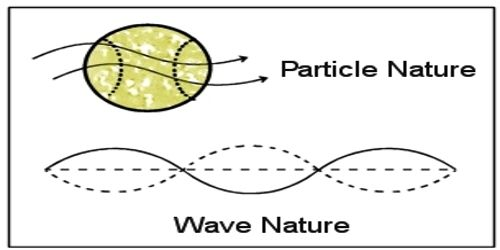Physics

# de Broglie’s Matter Wavesde Broglie’s Matter Waves

When a piece of red-hot iron is left somewhere we see radiation is emitted from it. Again, at night when we focus a torchlight, it illuminates as far as light spreads. This radiation and light emission apparently appears to be a continuous phenomenon. From Max Planck’s theory and later on from Einstein’s theory it is known that energy or radiation emitted from a body is not a continuous phenomenon. Energy or radiation is discrete i.e., energy is emitted as a bunch of packet or quanta.

From quantum theory it has been proved that radiation or energy has dual properties – one is particle property and the other is wave property. Within 23 years after the discovery of this theory no scientist thought about them that like energy a body can have two properties i.e., a body can also have wave property. In 1924 French scientist Luis de-Broglie first proposed this hypothesis. He mentioned that a material which is composed of different particles like atoms, molecules, proton, neutron, electron etc., will definitely demonstrate the wave property in appropriate conditions. In short, it can be said that matter has also dual nature like a wave. So, the wave is associated with each moving particle of matter. According to the name of the discoverer this wave is known as de Broglie’s matter waves and the wavelength of this wave is called de Broglie’s wavelength. The de Broglie–Bohm theory, also known as the pilot wave theory, Bohmian mechanics, Bohm’s interpretation, and the causal interpretation, is an interpretation of quantum mechanics.

Explanation: According to Planck’s quantum theory energy of a photon is,

E = hv … … … (1)

Here, h = Plank’s constant, v = frequency of the photon. If the mass of a photon particle is m, then according to Einstein’s mass-energy relation,

E = mc2 … … … (2)

Here, c = velocity of light. It is to be mentioned that photon moves with the velocity of light.

From equations (1) and (2) we get,

E = mc2 = hv

Suppose the momentum of photon = p

So, p = mass of a photon x velocity of the photon

= mc = hv/c2 x c = hv / c … … … (3)

Again, c = λv or, λ = c/v … … … (4)

From equation (3) and (4) we get,

p = hv/c = hv  /λv = h/λ

or, λ = h/p … … … (5)

In this equation dual properties of radiation has been expressed i.e., a relation has been established between the particle property of momentum and wave property of wavelength.

Now according to de Broglie’s hypothesis, if small particle like the electron is considered as photon particle, then its wavelength like photon will be,

λ = h/p = h / mv

Here, m = mass of the particle, v = velocity of the particle

and, mv = momentum of the particle.

This is the famous de-Broglie’s equation. The wave property of particles of matter has been expressed by it. From this equation, the wavelength of moving body can be determined.